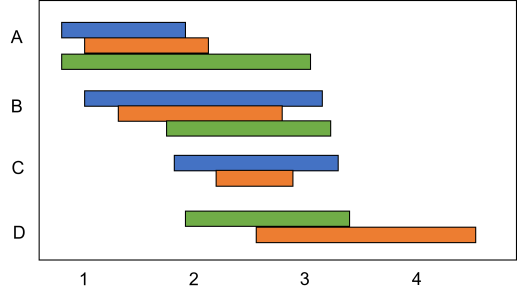# Floating bar plotHi,

I am trying to figure out how to make a floating bar plot (where each column has a range of values).  I don't find this option in Category plot... Any idea how to do this?

I sketch the figure below to exemplify what I aim to do.

Thanks!

C.I'm not sure if there is a way to do this directly. When I have needed to do something similar, I have coded up a 2D wave with x and y columns that have: start and stop coordinates followed by a nan row for each "bar". I used "lines between points" (with lsize >2). Colours can be specified in a second wave, and using f(z) to change the display.

Here is an example:

Make/O/T cat={"A", "B", "C"}
Make/O/D myOffset1={3,4,2}
Make/O/D myData1={4,4,2}
Make/O/D myOffset2={1,2,1}
Make/O/D myData2={4,3,2}

Window Graph0() : Graph
Display /W=(661.5,96.5,1056,305) myData1,myOffset1,myData2,myOffset2 vs cat
ModifyGraph rgb(myData1)=(0,15872,65280),rgb(myOffset1)=(65535,65535,65535),rgb(myOffset2)=(0,43520,65280)
ModifyGraph hbFill(myOffset1)=0,hbFill(myOffset2)=0
ModifyGraph toMode(myData1)=3,toMode(myData2)=3
ModifyGraph useBarStrokeRGB(myOffset1)=1,useBarStrokeRGB(myOffset2)=1
ModifyGraph barStrokeRGB(myOffset1)=(65535,65535,65535),barStrokeRGB(myOffset2)=(65535,65535,65535)
SetAxis/A/E=1 left
ModifyGraph swapXY=1
EndMacro

The resultant graph is attached.

The trick in this example is to use one wave for the lower value of the floating bar, and another for the length of the bar. Then in the category plot, choose 'stack on next' in the grouping options.

Hope this helps.

A variation on this that avoids the need to make the difference wave is to use grouping mode "Draw to Next":

make junk1= {0,6,2,3,4}
make junk2= {1,3,5,7,9}
make/t labels= {"one","two","three","four","five"}

Window Graph0() : Graph
PauseUpdate; Silent 1       // building window...
Display /W=(35,45,465,418) junk1,junk2 vs labels
ModifyGraph toMode(junk1)=1
ModifyGraph hideTrace(junk2)=2
SetAxis/A/E=1 left
EndMacro

Note two things: toMode = 1 for the FIRST trace, and the second trace is hidden.

I also set the left axis to "Autoscale from Zero", and failed to do the SwapXY thing, which is required to get horizontal bars.